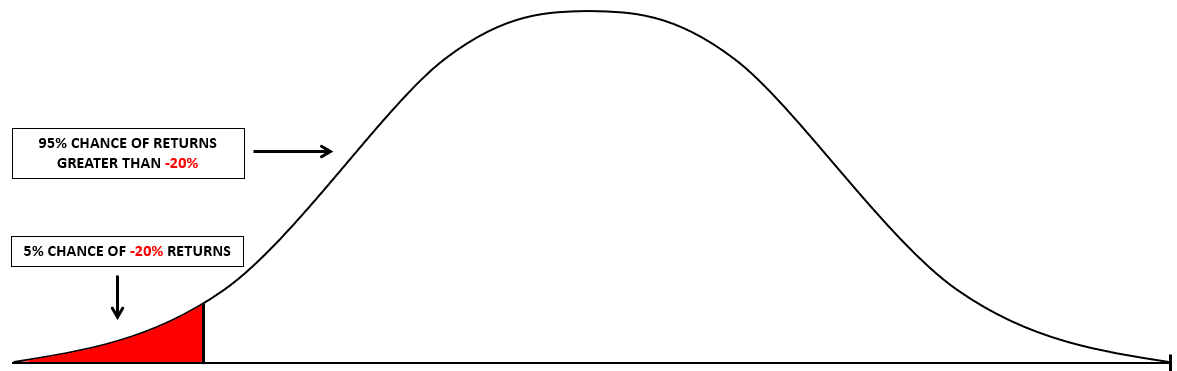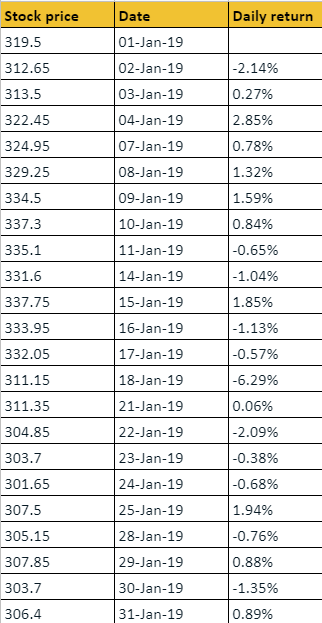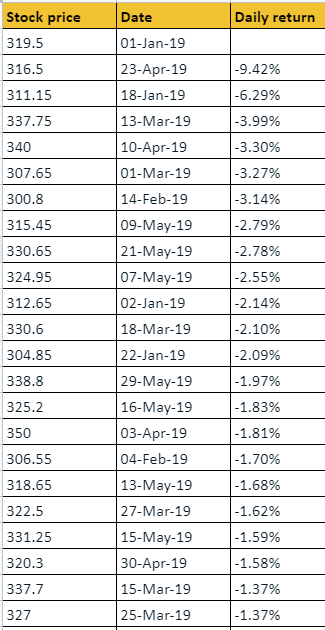# Introducing Value at Risk (VAR)

4.8Most compilations of investment advice will have this common line that you may have heard of - ‘Never invest more than you can afford to lose.’ That is based on the assumption that in the worst case scenario, you will end up losing all the money you invest. So, for example, if you have Rs. 10,000 available as spare cash, but can really only afford to part with about Rs. 4,000 (because you need the remaining Rs. 6,000 for necessary expenses), then it’s advisable to start your investments with Rs. 4,000. This is particularly true if you are investing in high-risk assets.

But, when you come to think of it, the term ‘more than you can afford to lose’ is quite vague, in some cases. It would be more helpful if you knew exactly how much you could stand to lose from a certain asset. That is where the concept of Value at Risk (VAR) comes into the picture.

### What is Value at Risk?

Value at Risk or VAR is a statistical measure of the level of financial risk within a portfolio, over a given period. It helps measure the amount of potential loss that your portfolio could incur over a specified investment tenure. So, you can estimate the probability of losing more than a given amount in a given portfolio.

This is important because once you know the probable losses, you can chart out your investment plan accordingly. It helps remove a great deal of the uncertainty associated with market-linked investments.

### Understanding Value at Risk

Up until now, we’ve been looking at volatility as a measure of risk. So, if measuring volatility can help you assess the risk, what is the need for VAR? You see, volatility refers to price movements on either side. If the price moves in the direction you expected, you earn returns on your investment. And if it moves in the opposite direction, you make losses. This is what risk represents.

So, risk and returns are two sides of the same coin. If an asset’s prices are highly volatile, it indicates that the price swings can move significantly on either side. This increases the possibility of higher returns, while also simultaneously increasing the risks. That’s where the high-risk-high-return category comes from.

While volatility is neutral to the direction of price movement, VAR is not. It specifically deals with the loss-making portion of price movements. It tackles the question of what your worst-case scenario is. After all, as an investor, isn’t that one of the most fundamental questions you need to answer before you invest in an asset?

### The components of VAR

VAR, especially in the parametric method, operates on the assumption that the prices (and the returns) of assets follow a normal distribution pattern. That makes all statistical analysis processes easier. So, the concept of Value at Risk has three key components:

1. The period of time over which the VAR is assessed
2. The level of confidence with which the assessment is made
3. The percentage/amount of loss

Putting these components together, VAR can be used to answer questions like the following:

• How much of my investment will I likely lose over the next year, with a 95% level of certainty?
• If I invest Rs. 1 lakh in a set of stocks, how much of my investment will I lose over the next half year, with a 99% level of confidence?

These are the kinds of questions VAR helps investors answer. The solution to these queries will help determine the possible losses. For example, using VAR, you may find out that in your portfolio, there is a 5% chance of losing 20% of your investments over the next year. So, this again has the same three components:

1. The period of time over which the VAR is assessed (here, 1 year)
2. The level of confidence with which the assessment is made (here, 95%)
3. The percentage/amount of loss (20% of your investments)

This is how the normal distribution curve for the above-mentioned scenario looks.### How can you calculate the Value at Risk?

There are three methods that you can use to assess the VAR. We’ll take a quick look at these methods now.

### 1. The historical method

The historical method is the simplest of the three techniques. It relies on the assumption that in the market, history tends to repeat itself. Here’s a step-by-step guide to this method. Let’s assume you’re taking historical returns data for a stock over a 100-day period.

• Step 1: List out the returns from the stock over the 100-day period.
• Step 2: Sort the data so it is arranged in an ascending order.
• Step 3: Choose the percentage of certainty with which you want to know the value at risk and determine the VAR.

Let’s look at an example to make this clearer. Say we take the stock price of Bharti Airtel Limited for the period from January 1, 2019 to May 29, 2019. This is a 101-day period, and when we calculate the returns on a day-by-day basis, we get 100 data points (for the period from January 2, 2019 to May 29, 2019).

Step 1: List out the returns from the stock over the 100-day period

So, the first step is to list out the returns in chronological order. Here’s a glimpse of the first part of the list.Step 2: Sort the data so it is arranged in an ascending order

Now, when we sort out the data in ascending order, this is how the first part of the list looks. Keep in mind that there are 100 data points here.See how the returns in the ‘daily return’ column are sorted in an ascending order. Like this, we have 100 data points ranging from -9.42% to 8.13%, which is the highest return in this data set.

Step 3: Choose the percentage of certainty with which you want to know the value at risk

Now, say you want to know what the worst-case scenario is. Naturally, that will be the lowest possible return, which, in this case, is -9.42%. But say you want to know the value at risk 5% of the time. That would go up to the 5 lowest returns you can get from this data set. In this case, as you can see, it is up to -3.27%. That is the fifth entry.

### 2. The variance-covariance method

The historical method is not always reliable, because year after year, different factors trigger the markets. For instance, taking historical data from 2019 for 2020 projections would not work, because the COVID-19 pandemic impacted the markets differently in 2020. Similarly, taking data from 202o for projecting the markets in 2021 would also not be the ideal situation.

Here’s where the variance-covariance method, also known as the parametric method, comes into the picture. For this, you only need to have two points of data:

1. The expected or average returns from a stock or a portfolio, as the case may be
2. The standard deviation of a stock or a portfolio, as the case may be

Once you have these two bits of information, you can make use of the empirical rule to identify the VAR with varying levels of certainty. As you may have guessed by now, the parametric method assumes that the data is normally distributed.

### 3. Monte Carlo simulation

This is a very tedious technique that involves randomly assigning different scenarios. Then, the worst cases of losses are identified. Needless to say, this can be impractical for large data sets. That’s why the parametric method is the most commonly used technique.

### How can the concept of VAR be helpful to you?

VAR is an important concept for every investor. If you are about to invest a sum in any asset, the VAR gives you a good idea of how much you could lose, in the worst case scenario. For conversative investors, this can be an excellent yardstick to identify the potential outcomes of their investments.

The great thing about VAR is that it can be applied to all kinds of assets - stocks, bonds, currencies, commodities, gold, real estate, anything really. It is universally applicable, making the VAR a good metric to measure up investments against. Risk management becomes easier with a tool like Value at Risk.

#### Wrapping up

Speaking of risk management, what is it anyway? Does it mean you avoid risk entirely? Or does it mean you try to reduce the risk in your investment portfolio. If that’s what you’re wondering, the next chapter holds the answers you’re looking for.

#### A quick recap

• Value at Risk or VAR is a statistical measure of the level of financial risk within a portfolio, over a given period.
• It helps measure the amount of potential loss that your portfolio could incur over a specified investment tenure.
• Volatility refers to price movements on either side.
• If the price moves in the direction you expected, you earn returns on your investment. And if it moves in the opposite direction, you make losses. This is what risk represents.
• VAR operates on the assumption that the prices (and the returns) of assets follow a normal distribution pattern. That makes all statistical analysis processes easier.
• There are three methods you can use to calculate VAR: the historical method, the parametric or the variance-covariance method, and the Monte Carle simulation method.
• The historical method is the simplest of the three techniques. It relies on the assumption that in the market, history tends to repeat itself.
• The variance-covariance method, also known as the parametric method, relies on two points of data: the expected or average returns and the standard deviation.
• This method assumes that the data is normally distributed.
• The Monte Carlo simulation is a very tedious technique that involves randomly assigning different scenarios. Then, the worst cases of losses are identified.